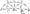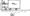# July 2011

Attempt any five questions.

Use of following supporting material is permitted during examination. (Mentioned in form No, 205)

1 (a) Write Area Moment Theorems (Mohr’s Theorems)

(b) A simply supported beam of length ‘L’ carries a central point load ‘W. If the moment of inertia is 3 I of the left half and

1 at the right half, find the slope at the supports and deflection at the midspan.

OR

1 (a) Derive the differential equation of a deflected beam as given d2y

by E=M where M is Bending Moment, E is Young’s dx mod. of elasticity and I is moment of inertia.

(b) A cantilever of length L is loaded with u.d.l. through out its length :

(i)  Calculate the deflection at a distance ‘C! from the free end.

(ii) If free end is propped, calculate the prop reaction.

(iii) If free end is propped, calculate the slope at prop end. Use area-moment theorems for solution.

2. A fixed beam of span 4 m carries u.d.l. of intensity 15 kN/m upto midspan. Calculate the fixed end moments and draw the S.F.D and B.M.D.

OR

2    Using Clapeyron’s theorem of three moments, draw the S.F.D. and B.M.D. of the continuous beam ABCD, simply supported at A, B and C and the end D is free, the spans are AB = 4m, BC = 4 m and CD = 2 m. The span AB carries a point load of 5 kN at the mid span, BC carries u.d.l. of 3 kN/m and CD carries a point load of 2 kN at free end D.

3. (a) A boiler is subjected to an internal pressure of 2 N/mm2, The thickness of the plate is 20 mm and allowable tensile stress is 120 N/mm2. Efficiency of the longitudinal joint is 90% and that of circumferential joint is 40%. Find out the maximum permissible diameter of the shell.

(b) A solid shaft is required to transmit 750 kW at 60 r.p.m. If the maximum value of shear stress is not to exceed 50 N/mm2, calculate the diameter of the shaft. If this solid shaft is replaced by a hollow shaft of diameter ratio 0.6, what will be the percentage saving of material ? The torque, maximum shear stress, the material and the length of shafts are some in either cases.

OR

3 (a) In a close-coiled spring, the diameter of each coil is to be 10 times that of wire of the spring and the maximum shear stress is not to exceed 60 N/mm2. Maximum permissible deflection under a load of 400 N is 100 mm. Taking the shear modulus as 9 x 104 N/mm2, determine the number of coils, the diameter of the coil and energy stored in the coil.

(b) A composite concentric shaft is made up of a solid steel shaft of 20 mm radius encased in a hollow steel tube of 30 mm inner radius and 50 mm outer radius. If both the shafts are of length I’m find the shear stress induced in both shafts and angle of twist. Take applied torque T – 1 kNm and shear modulus as 1 x 105 MPa.

4. (a)Determine the reactions at the supports for a two span continuous truss of fig. 1. Figures against the members are the areas of the respective members m members. mm2. Consider E as constant for all

OR

(a) A trussed timber beam, 4 m long, 120 mm wide and 250 mm deep has a central cast iron strut with an area of 2500 mm2 and a length of 625 mm. The steel tie rods are 28 mm in diameter. The beam carries a uniformly distributed load of 20 kN/m. Calculate the pull in the tie rods, the thrust in the strut and maximum stress in the beam section. Consider modulus of elasticity for timber, cast iron and steel as 1.2 x 10^ N/mm“, 10 x 10^ N/mm2 and 20 x 104 N/mm2 respectively, (fig. 2).AD and CD are of steel.

Fig. 2

5. (a)  Derive the differential equation for free vibration of undamped single degree of freedom systems. Also give the solution of the differential equation and define natural frequency of system.(b) Define the terms :

(i) critical damping

(ii) logarithmic decrement.

OR

(a) State the D’Almbert’s principle. Explain its application with example.

(b) What do you understand by degrees of freedom? How many degrees of freedom can be taken in each case in the figure given below for simplicity ?

\$-*y